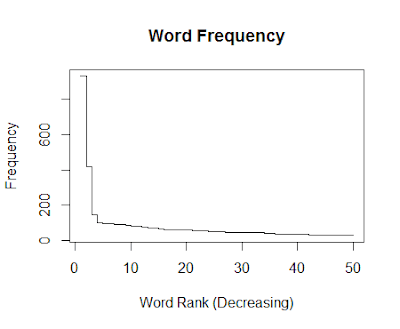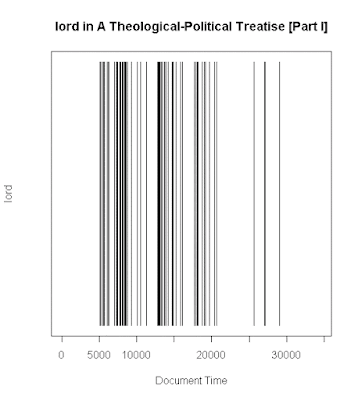### Plotting Text Frequency and Distribution using R for Spinoza's A Theological-Political Treatise [Part I]

This was a little bit of fun, after reading a few more chapters of Text Analysis with R for Students of Literature. Spinoza is a current interest, as I am also reading Radical Enlightenment: Philosophy and the Making of Modernity 1650-1750.

Example Code (Common to Subsections)

``````
# Text for this can be acquired as below Project Gutenberg, as below
# http://www.gutenberg.org/cache/epub/989/pg989.txt,
# or via a Sample Data at the end of this post:

# Text for this can be acquired via Matthew Jockers site, as below,
# http://www.matthewjockers.net/macroanalysisbook/expanded-stopwords-list/,
# or via a Sample Data at the end of this post:
exclusionFile = 'StopList_Extended.csv'

text.scan <- scan(file = textToRead, what = 'char')
text.scan <- tolower(text.scan)

# Create list
text.list <- strsplit(text.scan, '\\W+', perl = TRUE)
text.vector <- unlist(text.list)

# Create exclusion list
exclusion.file <- scan(exclusionFile, what='char')
exclusion.v <- strsplit(exclusion.file, ',', perl = TRUE)
exclusion.v <- tolower(exclusion.v)

# Remove exclusions
not.exclusions.v <- which(!text.vector %in% exclusion.v)
text.vector <- text.vector[not.exclusions.v]

# Create sorted list
text.frequency = table(text.vector)
text.frequency.sorted = sort(text.frequency, decreasing = TRUE)
``````

Example Code for Frequency Plot

``````
# Plots
# Types: p = point, l = line, b = both, h = hist, s = stairs
plot(text.frequency.sorted[1:50]
, type="s"
, main = "Word Frequency"
, xlab = "Word Rank (Decreasing)"
, ylab = "Frequency")``````

Example Code for Dispersion Plots

``````
n.time.v = seq(1:length(text.vector))

textToPlot = 'Spinoza\'s A Theological-Political Treatise [Part I]'
wordToPlot = 'god'
wordToPlot = 'law'
wordToPlot = 'prophets'
wordToPlot = 'lord'

word.v <- which(text.vector == wordToPlot)
w.count.v <- rep(NA, length(n.time.v))
w.count.v[word.v] <- 1

plot(w.count.v
, main = paste('Dispersion Plot of', wordToPlot, 'in', textToPlot, sep = " ")
, xlab = "Document Time"
, ylab = wordToPlot
, type = "h"
, ylim = c(0, 1), yaxt = 'n')``````
Sample Data